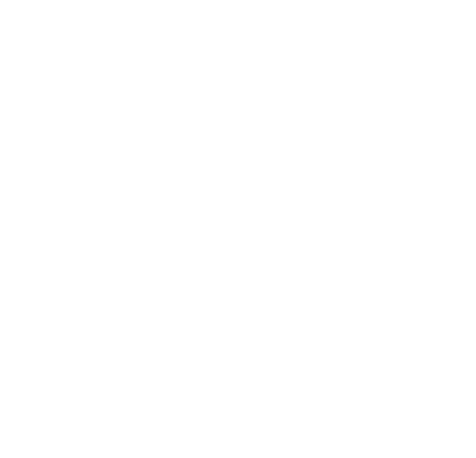Open in App
Not now

# How to draw color filled star in Python-Turtle?

• Last Updated : 08 Oct, 2020

Turtle is an inbuilt module in Python. It provides drawing using a screen (cardboard) and turtle (pen). To draw something on the screen, we need to move the turtle (pen). To move the turtle, there are some functions i.e forward(), backward(), etc.

Approach:

The following steps are used:

• Import turtle
• Set window screen
• Set color of the turtle
• Form a star
• Fill the star with the color

Below is the implementation.

## Python3

 `# importing package``import` `turtle` `# function to draw``# colored star``def` `colored_star():``    ` `    ``# size of star``    ``size ``=` `80``    ` `    ``# object color``    ``turtle.color(``"red"``)``    ` `    ``# object width``    ``turtle.width(``4``)``    ` `    ``# angle to form star``    ``angle ``=` `120``    ` `    ``# color to fill``    ``turtle.fillcolor(``"yellow"``)``    ``turtle.begin_fill()``    ` `    ``# form star``    ``for` `side ``in` `range``(``5``):``        ``turtle.forward(size)``        ``turtle.right(angle)``        ``turtle.forward(size)``        ``turtle.right(``72` `-` `angle)``        ` `    ``# fill color``    ``turtle.end_fill()` `# Driver code``colored_star()`

Output :Since, as of now, you must have learned how to draw and fill color in a star. So, let’s try something unique with this knowledge. Let’s use some creativity and programming to build a night full of stars.

## Python3

 `import` `turtle``from` `random ``import` `randint` `window ``=` `turtle.Screen()``turtle.bgcolor(``"black"``)``turtle.color(``"yellow"``)``turtle.speed(``0``)` `def` `draw_star():``    ``turns ``=` `6``    ``turtle.begin_fill()``    ``while` `turns>``0``:``        ``turtle.forward(``25``)``        ``turtle.left(``145``)``        ``turns ``=` `turns``-``1``    ``turtle.end_fill()` `nums_stars ``=` `0``while` `nums_stars <``50``:``    ``x``=``randint(``-``300``,``300``)``    ``y``=``randint(``-``300``,``300``)``    ``draw_star()``    ``turtle.penup()``    ``turtle.goto(x,y)``    ``turtle.pendown()``    ``nums_stars ``=` `nums_stars ``+` `1` `window.exitonclick()`

Output:

My Personal Notes arrow_drop_up